# Utility class for generating the k-subsets of the numbers 0 to n : Algorithms « Collections Data Structure « Java

Utility class for generating the k-subsets of the numbers 0 to n

```
/*
* Created on 05-Aug-2005 by Ryan McNally
*/

//package com.ryanm.util.sets;

/**
* Utility class for generating the k-subsets of the numbers 0 to n
*
* @author ryanm
*/
public class SubsetGenerator {
private final int desiredSubsetSize;

private final SubsetIterator iterator;

private int[] nextSubset = null;

/**
* Constructs a new {@link SubsetGenerator}
*
* @param setSize
*            The size of the set
* @param subsetSize
*            The desired size of the subsets
*/
public SubsetGenerator(int setSize, int subsetSize) {
desiredSubsetSize = subsetSize;
iterator = new SubsetIterator(setSize, subsetSize);

while (iterator.hasNext()
&& (nextSubset == null || nextSubset.length != desiredSubsetSize)) {
int[] a = iterator.next();
nextSubset = a;
}
}

/**
* Gets the next subset
*
* @return A subset
*/
public int[] next() {
int[] results = nextSubset;

nextSubset = null;
while (iterator.hasNext()
&& (nextSubset == null || nextSubset.length != desiredSubsetSize)) {
int[] a = iterator.next();
nextSubset = a;
}

if (nextSubset.length != desiredSubsetSize) {
nextSubset = null;
}

return results;
}

/**
* Determines if there are any more subsets to be had
*
* @return <code>true</code> if there are more subsets, <code>false</code>
*         otherwise
*/
public boolean hasNext() {
return nextSubset != null;
}

private class SubsetIterator {

private boolean hasNext;

private boolean jump = false;

private int k = 0;

private int maxSize, n, is;

private int[] a;

/**
* Constructor.
*
* @param n
*            number of elements of the set.
* @param maxSize
*            maximal size of subset.
*/
private SubsetIterator(int n, int maxSize) {
if (n < 0) {
throw new IllegalArgumentException("n < 0");
}

// if( maxSize < 0 || maxSize > n )
// throw new IllegalArgumentException( "maxSize < 0 ||
// maxSize > n" );

a = new int[maxSize];
this.maxSize = maxSize;
this.n = n;
hasNext = n != 0 && maxSize > 0 && maxSize <= n;
}

/**
* Determines whether there are any more subsets to come
*
* @return true if there are more subsets, false otherwise
*/
public boolean hasNext() {
return hasNext;
}

/**
* Returns the next subset of the n-set. Each subset is represented by
* an integer array which elements are listed in increasing order.
*
* @return an array with the elements of the original set present in the
*         subset.
* @throws IllegalStateException
*             there are no more subsets.
*/
public int[] next() {
if (!hasNext) {
throw new IllegalStateException("no next");
}

if (k == 0) {
if (!jump) {
is = 0;
k++;
}
} else {
if (a[k - 1] == n - 1) {
k--;

if (k == 0) {
return getResultSet();
}

is = a[k - 1] + 1;
} else {
is = a[k - 1] + 1;
if (!jump) {
k++;
}
}
}

a[k - 1] = is;

jump = k == maxSize;
if (a == n - 1) {
hasNext = false;
}

return getResultSet();
}

/**
* Returns the array with the result.
*
* @return the array with the result.
*/
private int[] getResultSet() {
int[] result = new int[k];
System.arraycopy(a, 0, result, 0, k);
return result;
}
}
}

```

### Related examples in the same category

 1 Anagrams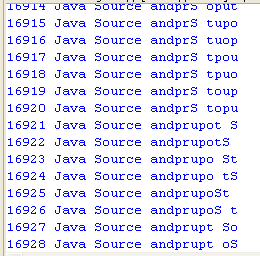2 Hanoi puzzle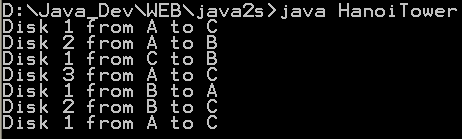3 Fibonacci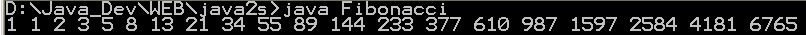4 Sieve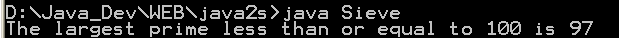5 Find connections using a depth-first search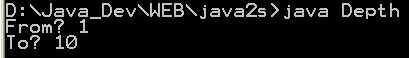6 Find connections using hill climbing. 7 Find optimal solution using least-cost 8 Find the lost keys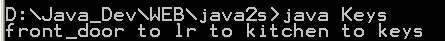9 Compute the area of a triangle using Heron's Formula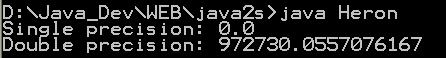10 Compute prime numbers 11 Print a table of fahrenheit and celsius temperatures 1 12 Print a table of fahrenheit and celsius temperatures 2 13 Print a table of Fahrenheit and Celsius temperatures 3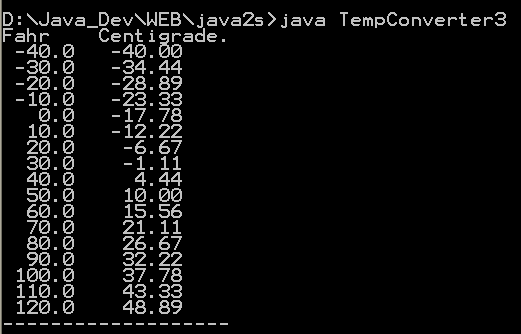14 Soundex - the Soundex Algorithm, as described by Knuth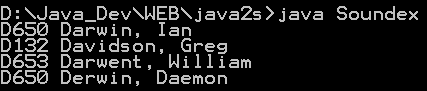15 A programmable Finite State Machine implementation. 16 An extendable Graph datastructure. 17 Utilities for flop (floating-point operation) counting. 18 LU Decomposition 19 Reverse Polish Notation 20 Permutator test 21 implements the LZF lossless data compression algorithm 22 Linear Interpolation 23 Version